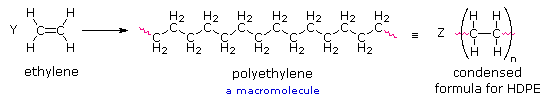# Writing Formulas for Polymers

$$\newcommand{\vecs}{\overset { \rightharpoonup} {\mathbf{#1}} }$$ $$\newcommand{\vecd}{\overset{-\!-\!\rightharpoonup}{\vphantom{a}\smash {#1}}}$$$$\newcommand{\id}{\mathrm{id}}$$ $$\newcommand{\Span}{\mathrm{span}}$$ $$\newcommand{\kernel}{\mathrm{null}\,}$$ $$\newcommand{\range}{\mathrm{range}\,}$$ $$\newcommand{\RealPart}{\mathrm{Re}}$$ $$\newcommand{\ImaginaryPart}{\mathrm{Im}}$$ $$\newcommand{\Argument}{\mathrm{Arg}}$$ $$\newcommand{\norm}{\| #1 \|}$$ $$\newcommand{\inner}{\langle #1, #2 \rangle}$$ $$\newcommand{\Span}{\mathrm{span}}$$ $$\newcommand{\id}{\mathrm{id}}$$ $$\newcommand{\Span}{\mathrm{span}}$$ $$\newcommand{\kernel}{\mathrm{null}\,}$$ $$\newcommand{\range}{\mathrm{range}\,}$$ $$\newcommand{\RealPart}{\mathrm{Re}}$$ $$\newcommand{\ImaginaryPart}{\mathrm{Im}}$$ $$\newcommand{\Argument}{\mathrm{Arg}}$$ $$\newcommand{\norm}{\| #1 \|}$$ $$\newcommand{\inner}{\langle #1, #2 \rangle}$$ $$\newcommand{\Span}{\mathrm{span}}$$$$\newcommand{\AA}{\unicode[.8,0]{x212B}}$$

The repeating structural unit of most simple polymers not only reflects the monomer(s) from which the polymers are constructed, but also provides a concise means for drawing structures to represent these macromolecules. For polyethylene, arguably the simplest polymer, this is demonstrated by the following equation. Here ethylene (ethene) is the monomer, and the corresponding linear polymer is called high-density polyethylene (HDPE). HDPE is composed of macromolecules in which n ranges from 10,000 to 100,000 (molecular weight $$2 \times 10^5$$ to $$3 \times10^6$$ ).If Y and Z represent moles of monomer and polymer respectively, Z is approximately $$10^{-5}$$ Y. This polymer is called polyethylene rather than polymethylene, $$\ce{(-CH_2-)_{n}}$$, because ethylene is a stable compound (methylene is not), and it also serves as the synthetic precursor of the polymer. The two open bonds remaining at the ends of the long chain of carbons (colored magenta) are normally not specified, because the atoms or groups found there depend on the chemical process used for polymerization. The synthetic methods used to prepare this and other polymers will be described later in this chapter.

## Contributors

William Reusch, Professor Emeritus (Michigan State U.), Virtual Textbook of Organic Chemistry

Writing Formulas for Polymers is shared under a CC BY-NC-SA 4.0 license and was authored, remixed, and/or curated by LibreTexts.